# 等下一个花季

## 正文第十章 项链

[更新时间] 2019-03-16 16:11:38 [字数] 3298

宋小冉叫她去超市的时候尹天稚正在发呆，两眼放空，眼神呆滞，宋小冉叫了她好几声都没有回应。宋小冉只好走到她身边吼了一句：“想什么呢？”*=@=-本书首-发纵横%中=文网-=@!|

差点把尹天稚的小心脏吓到停止跳动，不安的拍着自己的胸膛。*=@=-本书首-发纵横%中=文网-=@!|

“你吓死我了。”*=@=-本书首-发纵横%中=文网-=@!|

人吓人真的会吓死人好么。*=@=-本书首-发纵横%中=文网-=@!|

宋小冉一屁股坐在了尹天稚的桌子上俯视她，有点傻乎乎的。“去不去超市啊？”*=@=-本书首-发纵横%中=文网-=@!|

“你等会，我拿一下卡。”*=@=-本书首-发纵横%中=文网-=@!|

说完尹天稚就伸手到抽屉盒里拿出上衣外套，拿到卡的同时尹天稚却发现呵卡放在一个兜里的项链却不见了。她连忙重新搜索了一遍，就差把衣服翻出个洞了。就连抽屉里翻了个遍，结果尹天稚悲催的发现：*=@=-本书首-发纵横%中=文网-=@!|

项链不见了。*=@=-本书首-发纵横%中=文网-=@!|

见到这幅情景的宋小冉也察觉到情况不对，脸上立马露出了担心的表情，关心地问尹天稚：“怎么了吗，找什么呢？”*=@=-本书首-发纵横%中=文网-=@!|

尹天稚急得想骂人，那条项链对她非常重要，她不能接受这个事实。即使这样，尹天稚还是平静下来对宋小冉说：“我的项链不见了。”*=@=-本书首-发纵横%中=文网-=@!|

看见尹天稚着急，宋小冉也为她着急。*=@=-本书首-发纵横%中=文网-=@!|

“你好好想一想去过什么地方，有没有可能掉在哪里？”*=@=-本书首-发纵横%中=文网-=@!|

经过宋小冉这么一提醒，尹天稚脑海中立马冒出了一个可怕的念头——男厕所，一想到这，她浑身的鸡皮疙瘩都起来了，身子都在那一瞬间僵硬了。*=@=-本书首-发纵横%中=文网-=@!|

尹天稚立马冲了出去。*=@=-本书首-发纵横%中=文网-=@!|

“需要我帮你找吗？”宋小冉在身后喊到。*=@=-本书首-发纵横%中=文网-=@!|

尹天稚摇了摇手，“不用了，你和她们去超市吧，不用等我了。”她总不能告诉宋小冉自己要去闯男厕所吧。*=@=-本书首-发纵横%中=文网-=@!|

声音消失在教室门口，宋小冉有些无奈的摇了摇头。*=@=-本书首-发纵横%中=文网-=@!|

这丫头不仅蠢，还这么丢三落四的。*=@=-本书首-发纵横%中=文网-=@!|

现在正是人群上厕所的高峰期，男厕所门口的人群进进出出，进进出出，看得尹天稚头晕眼花。躲在小墙角默默的观察一切，尹天稚的小脑袋探出来看两眼有立马缩进去，那样子好不可爱。*=@=-本书首-发纵横%中=文网-=@!|

乔安不知道这是第几次来到男厕所了，一下课他就匆匆忙忙的过来了，为的就是逮住尹天稚那个疯女人狠狠地训她一顿。*=@=-本书首-发纵横%中=文网-=@!|

“乔安，上厕所这么频繁，你没事吧。”*=@=-本书首-发纵横%中=文网-=@!|

同行的人看了看他的下半身，有些不怀好意的嘲笑他。*=@=-本书首-发纵横%中=文网-=@!|

乔安捶了捶他的胸口，“上你的厕所吧，在外面等你。”*=@=-本书首-发纵横%中=文网-=@!|

“好嘞！”乔安用目光四处搜索着那个身影，正当他想放弃的时候，却在一个小角落里发现了那个鬼鬼祟祟的小脑袋。*=@=-本书首-发纵横%中=文网-=@!|

尹天稚刚探出头来的那一刻，四目相望，吓得尹天稚赶紧缩回脑袋，身体紧紧的贴在墙面上，一动也不敢动。*=@=-本书首-发纵横%中=文网-=@!|

乔安慢慢的走过去，故意让自己额脚步走的有规律并且发出稳健的脚步声，他就是要让尹天稚紧张，让她知道心虚这两个字是怎么写的。*=@=-本书首-发纵横%中=文网-=@!|

尹天稚能清晰的听到心里小鹿乱撞的声音，乔安的脚步越来越近，她心里也越来越不安。*=@=-本书首-发纵横%中=文网-=@!|

看不见我看不见我，怎么还不走，越来越近了怎么办？如果他知道了怎么办，怕什么，大不了打一场，谁输谁赢还不一定呢。况且他又不知道是我做的。*=@=-本书首-发纵横%中=文网-=@!|

打定了主意的尹天稚刚想出去，脚步声却戛然而止，尹天稚有些疑惑的探出头去。*=@=-本书首-发纵横%中=文网-=@!|

难道他走了？*=@=-本书首-发纵横%中=文网-=@!|

正这样想着，乔安却神不知鬼不觉的来到尹天稚面前，“墙角舒服吗？”*=@=-本书首-发纵横%中=文网-=@!|

尹天稚吓的三魂不见了七魄，用手拍着自己的小心脏压压惊。*=@=-本书首-发纵横%中=文网-=@!|

我的小心脏这几天都快承受不住负荷了好吗。*=@=-本书首-发纵横%中=文网-=@!|

“有病啊你，知不知道人吓人会吓死人的。”*=@=-本书首-发纵横%中=文网-=@!|

乔安凑近尹天稚，他的声音很好听，带有些许蛊惑的味道，身上的湿衣服已经换下了，刚刚那狼狈的样子早已不见。他轻轻的说“你又没有做亏心事，怕什么？”*=@=-本书首-发纵横%中=文网-=@!|

尹天稚的心跳突然露了一拍，直觉告诉她，乔安一定知道了。*=@=-本书首-发纵横%中=文网-=@!|

“我，我当然，没有。”尹天稚越说越小声，口齿也有些不清楚了。*=@=-本书首-发纵横%中=文网-=@!|

一条项链突然出现在眼前，乔安用手吊着项链慢慢的移到尹天稚的眼前，口气轻挑“这是谁的呢？”尹天稚就这样看着也不说话，乔安一把握住项链“不说话，嗯。”*=@=-本书首-发纵横%中=文网-=@!|

尹天稚很纠结，她要是承认的话就有可能被打，她要是否认的话还不知道会被乔安怎么刁难，所以她选择了沉默。*=@=-本书首-发纵横%中=文网-=@!|

我就不说话，你能怎么办？*=@=-本书首-发纵横%中=文网-=@!|

“好吧，既然没人要的话，我就把它丢到这墙外面去了。”说完乔安直起身子，作势就要把项链扔出去。*=@=-本书首-发纵横%中=文网-=@!|

尹天稚赶紧抱住了他的手，神情十分着急“别，别啊，有话好好说。”*=@=-本书首-发纵横%中=文网-=@!|

“怎么，要坦白吗？”*=@=-本书首-发纵横%中=文网-=@!|

尹天稚可怜巴巴的看着乔安，委屈的说“我错了，原谅我吧。”*=@=-本书首-发纵横%中=文网-=@!|

乔安被她这样的样子逗笑了，一排大白牙在阳光的照射下白的发亮，说实话他这样笑起来异常帅气。“叫什么名字？”*=@=-本书首-发纵横%中=文网-=@!|

“尹天稚。”小声地。*=@=-本书首-发纵横%中=文网-=@!|

“什么，听不清。”*=@=-本书首-发纵横%中=文网-=@!|

尹天稚踮起脚尖凑到乔安的耳朵旁大声的喊道“尹天稚！”*=@=-本书首-发纵横%中=文网-=@!|

乔安不舒服的揉了揉耳朵，差点耳朵都被她喊到失鸣。*=@=-本书首-发纵横%中=文网-=@!|

这丫头一定是故意的。*=@=-本书首-发纵横%中=文网-=@!|

乔安一低头果然看见了她嘴角擒着的笑意。*=@=-本书首-发纵横%中=文网-=@!|

乔安把项链递到尹天稚面前，看她高兴的就伸手来接，乔安却再次握住了项链。“这么宝贵它，不会是心上人送的吧？”*=@=-本书首-发纵横%中=文网-=@!|

尹天稚给了他一个白眼，这人就是想太多。*=@=-本书首-发纵横%中=文网-=@!|

“说什么呢，虽然不是心上人，但也是一个非常重要的朋友送的。”*=@=-本书首-发纵横%中=文网-=@!|

她现在一定长的和我一般高了。*=@=-本书首-发纵横%中=文网-=@!|

“哦，这样啊。”乔安似懂非懂的点点头，“答应我一件事就还给你。”*=@=-本书首-发纵横%中=文网-=@!|

尹天稚气呼呼的看着他，就知道没这么简单。*=@=-本书首-发纵横%中=文网-=@!|

“星期五在铭城公园有一场篮球赛，到时候来看吧。”*=@=-本书首-发纵横%中=文网-=@!|

尹天稚不可思议的盯着乔安，“就这样？”*=@=-本书首-发纵横%中=文网-=@!|

“就这样。”*=@=-本书首-发纵横%中=文网-=@!|

乔安把项链放在尹天稚的手中，潇洒的转身离开。*=@=-本书首-发纵横%中=文网-=@!|

“乔安，去哪了你？”同行的人向他招招手。*=@=-本书首-发纵横%中=文网-=@!|

“有点事，走吧，回去吧。”*=@=-本书首-发纵横%中=文网-=@!|

尹天稚有点懵，乔安居然只是让她去看一场篮球赛，有点不真实的感觉。*=@=-本书首-发纵横%中=文网-=@!|

真的就这么简单？*=@=-本书首-发纵横%中=文网-=@!|

在晴朗的夜空下，女生宿舍307窗前，尹天稚呆呆的坐在那里，望着手中的项链出神。路过的宋小冉停住脚步看着尹天稚手中的项链问：“这就是你丢的项链？”*=@=-本书首-发纵横%中=文网-=@!|

“嗯啊，是的。”*=@=-本书首-发纵横%中=文网-=@!|

宋小冉刚洗完脸，贴了片面膜在脸上。尹天稚想不到如此彪悍的她也会有这么女孩子的一面。*=@=-本书首-发纵横%中=文网-=@!|

“挺漂亮的，小心别丢了。”*=@=-本书首-发纵横%中=文网-=@!|

尹天稚爱惜的摸了又摸，嘴角是掩不住的笑意，耳边突然响起了那个稚嫩的声音。*=@=-本书首-发纵横%中=文网-=@!|

“尹天稚，带上了它我们就永远都是朋友了。即使你以后有了男朋友，我也会告诉他让他一边去，我要和你共白头，做一对快乐的老奶奶。”*=@=-本书首-发纵横%中=文网-=@!|

想着想着，尹天稚忍不住笑出声来，随后一抹愁绪又爬上眉头，久久不能平复。*=@=-本书首-发纵横%中=文网-=@!|

你在哪呢？还记得我吗？我还想和你共白头呢！*=@=-本书首-发纵横%中=文网-=@!|

米白走了过来，一把就抢走了尹天稚手中的项链，嘲笑道“宋小冉，即使你对首饰不敢兴趣，那也不能睁着眼睛说瞎话吧，这款式都过时了好吧。尹天稚，你的眼光也太差了吧。”*=@=-本书首-发纵横%中=文网-=@!|

一群乡巴佬。*=@=-本书首-发纵横%中=文网-=@!|

唐今竹也走了过来，很不满米白这样说话的方式，“米白你说话过分了啊，怎么说也是一个宿舍的，还是同学，好好处不行吗。”*=@=-本书首-发纵横%中=文网-=@!|

听到争吵声的苏溪也抬起头来，眼神扫过了在场的所有人，也没有上前制止，只是就这样默默地看着。*=@=-本书首-发纵横%中=文网-=@!|

看戏不嫌事大。*=@=-本书首-发纵横%中=文网-=@!|

米白听见唐今竹这么说，犀利的眼光就这么盯着她，让唐今竹有些心里发毛。“我就是实话实说，唐今竹你这个肥婆别多管闲事。”*=@=-本书首-发纵横%中=文网-=@!|

唐今竹气的眼眶泛红。*=@=-本书首-发纵横%中=文网-=@!|

尹天稚站起身来拿过自己的项链，小心翼翼的放在礼品盒保存起来。米白转而又把枪口对转她，“尹天稚，你要是没有首饰，我可以给你啊，我买了好多呢？”*=@=-本书首-发纵横%中=文网-=@!|

“你买的？”尹天稚话里有话的问。*=@=-本书首-发纵横%中=文网-=@!|

“是啊。”*=@=-本书首-发纵横%中=文网-=@!|

尹天稚绕着她转了一圈，把她浑身都看了个遍。“也对，像你这样的只能自己买项链，怎么能体会到别人送首饰的快乐呢。”*=@=-本书首-发纵横%中=文网-=@!|

米白有些生气，尹天稚继续挖苦她说：“哎呀，活成你这样还真是悲哀哈，只能自己买首饰。”*=@=-本书首-发纵横%中=文网-=@!|

“说什么呢你。”米白抬手就要给她一个耳光，尹天稚刚想反抗，有一个人反应更快，在米白的耳光落到尹天稚脸上的那一刻抓住了她的手。*=@=-本书首-发纵横%中=文网-=@!|

宋小冉对着米白恶狠狠的说：“动我的人，你是想死吗？”宋小冉的手一用力就痛的米白眉毛都挤在一起了，本来就不漂亮，现在更丑了。*=@=-本书首-发纵横%中=文网-=@!|

宋小冉猛的一推她，米白就这样跌坐在地上。*=@=-本书首-发纵横%中=文网-=@!|

尹天稚觉得宋小冉真是太帅了，全身的血液都在沸腾着，但尹天稚更在意的是那一句：我的人。*=@=-本书首-发纵横%中=文网-=@!|

你给的感动我也可以负担得起。*=@=-本书首-发纵横%中=文网-=@!|

尹天稚蹲在米白面前，她最忍受不了辱骂她的朋友，这是底线。“不要动不动就说人胖，你要知道胖是一时的，而丑是一世的。别以为你长得黑别人就不知道你是白痴，白痴。”*=@=-本书首-发纵横%中=文网-=@!|

米白怒了，但是又有些后怕的看了看宋小冉，只得硬生生的受着尹天稚的话，敢怒不敢言。*=@=-本书首-发纵横%中=文网-=@!|

尹天稚就是看不惯她这幅欺软怕硬的样子，不给她点颜色看看还以为自己好欺负呢。*=@=-本书首-发纵横%中=文网-=@!|

尹天稚搂着唐今竹的肩膀，挑衅的对着米白说：“都说胖子是潜力股，我们今竹要是瘦下来，指不定女神，对吧宋小冉。”*=@=-本书首-发纵横%中=文网-=@!|

宋小冉点了点头表示同意。*=@=-本书首-发纵横%中=文网-=@!|

唐今竹很感动，一直以来都被人嘲笑的她第一次感受到了温暖。虽然每次被人拿体重开玩笑都只能回以一笑，又有多少人知道，其实胖子很介意。*=@=-本书首-发纵横%中=文网-=@!|

三个人相视而笑，这一刻她们在一起面对，未来，她们也一起走。*=@=-本书首-发纵横%中=文网-=@!|

*=@=-本书首-发纵横%中=文网-=@!|

(快捷键：←)上一章 回目录(快捷键：回车) 下一章(快捷键：→)

### 发表评论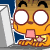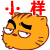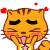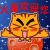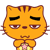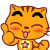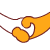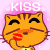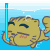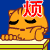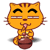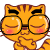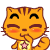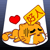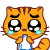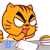12